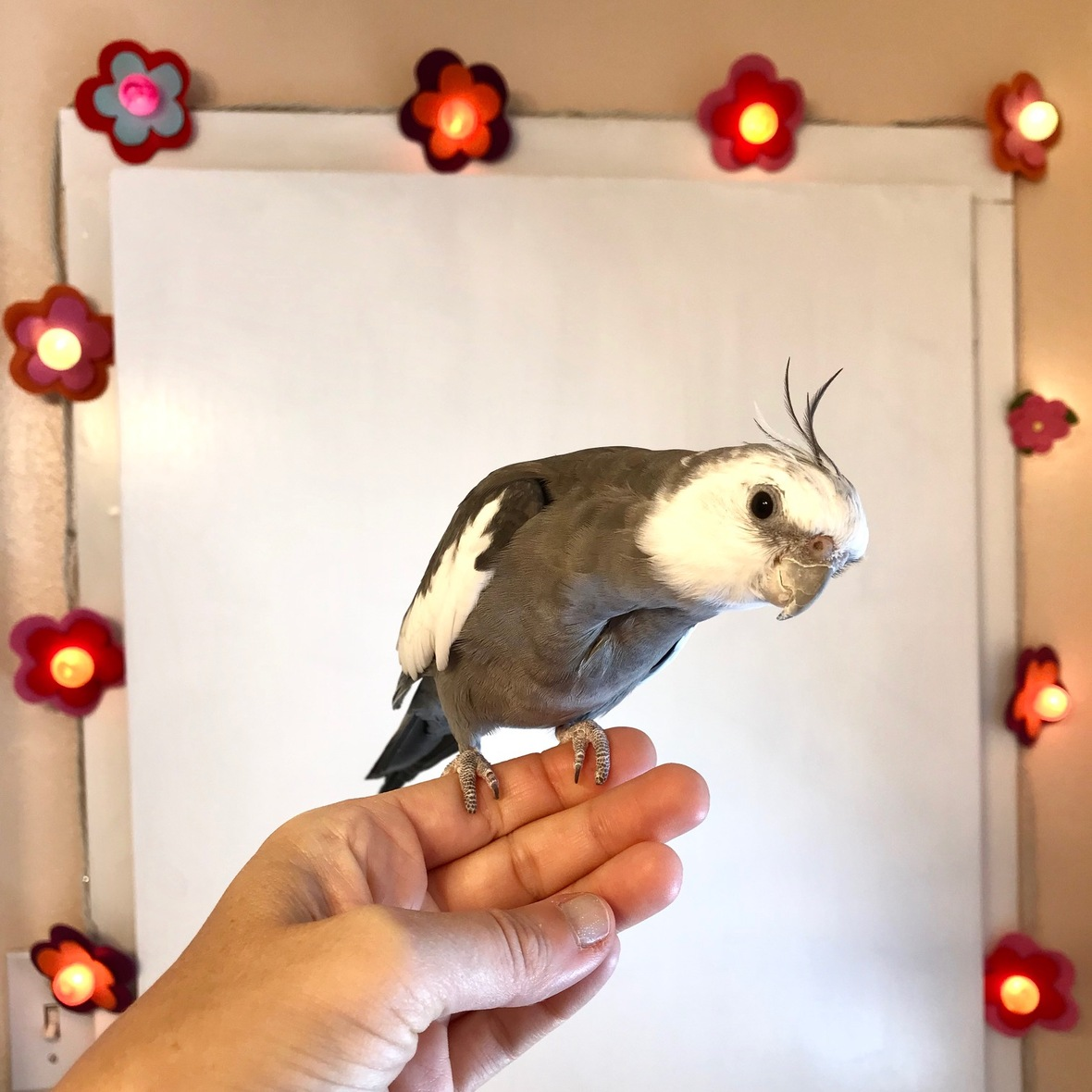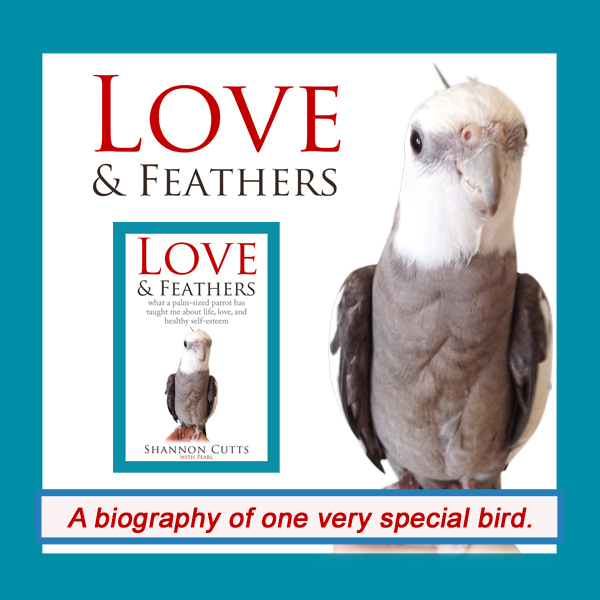# December 2018

 table div table+table+table div table{width:100%;padding:0}table div table+table+table div table img{width:96.23%;padding:0;float:none}table div table+table+table div table td{width:100%;padding:0 1.88% 18px}/* styles *//* styles */ Receiving this email as a forward? Sign up to keep following our little flock's adventures!
 table div table+table+table+table+table+table div table{width:100%;padding:0}table div table+table+table+table+table+table div table img{width:96.23%;padding:0;float:none}table div table+table+table+table+table+table div table td{width:100%;padding:0 1.88% 18px}/* styles */## Look Inside and See Who Is ThereIf there is one being who doesn't worry about looking in and seeing someone wonderful it is my precious parrot, Pearl! If I had to sum up his life motto, it might be "why wait for a new year when you can start celebrating your very pretty, feathery and fetching self right now?!"

So here we are. Here I am. Another year has raced (shuffled) by. Looking back might still feel premature....looking forward too vast, wide-open.

So we look within. I look within. I feel like we are all born with certain "presets" - personality traits or bents or preferences. Some of us are more outgoing and extroverted, while others (such as yours truly) are more introverted and solitary.

But then we also gather up the presets or preferences of others - our parents, teachers, peers, friends, frenemies - as we go through life. One person wants us to be more like this, while another person wishes we were more like that.

We probably try our best to accommodate. How hard we try depends a lot on how much we want what only seems possible by conforming, or how afraid we are of the consequences of not conforming, such as losing the company of someone we feel we love or want or need in our life.

But then there is us, ourselves, our own company. Maybe we are born loving the company we naturally keep. Maybe we start out that way (I truly believe we all do - whyever would we not?) and then gradually over time and with exposure to others we perhaps become disenchanted with ourselves. We wish we were different. We wish we looked different, acted different, spoke different, lived different.

We strive to make these changes. We think - I think - "well I'm here now, I have this one life to live, why not go for what I want in life?"

There is just one teensy, tiny issue. What I want isn't to change myself. What I want is to become more fully myself. I say this because today, at the end of another fast (slow) year of highlights and lowlights, I have learned one and only one truth, which is that I am precisely, perfectly, who I am supposed to be.

I'm just not always sure who that person is. Some days it feels like we are buddies, friends. Some days, we are on our first coffee date. Some days we pass each other in the grocery store aisle and don't even recognize each other. In rare moments, we bump into each other on our way to and from the bathroom and midnight (again) and, joyously, embrace. We fall asleep and dream we've met our best friend.

We wake up, alone again and missing someone we are afraid we will never ever meet.

Or at least I am afraid of this. I use the term "we" because I have a few treasured soul friends who have let me know I am not the only one who is afraid of this - of all these things. It is fearful-making, the thought (threat) of living a whole life inside the body, mind, heart of a stranger.

I do believe what one of my most treasured mentors once said as she told a story,

There are two people arguing. They are having the most heated discussion, each entrenched in their perspective and position. They are both so angry! But then, on the inside, deep down where neither person can see, their two spirits are smiling and laughing together and having a good time!

I do feel this way sometimes, in my rare wiser moments when, in the midst of loss or anger or separation I can sense my spirit telling me, "Don't worry - everything really is all right."

In those moments, I feel comforted, not from others but from within. I feel I am among, within, friends.

But many days, when I wake up each morning and power up my #yogawithadriene session online or when I start my morning meditation or when I head out for a bike ride or do my journal contemplations, I look inside and don't see anyone I recognize.

And I wonder....will I ever know her? Will I ever truly get to know the one true being that is me? Will there be one day, and then a series of days, and then weeks and months and then years when I look inside and see who is there, and I SEE her....I SEE ME.

This is the year of seeing - of looking inside and seeing who is there. Of seeing her as she is. Of seeing me as I am. This is the year I feel I have been looking forward to all my life.

I sure would love some good company to join me on this journey (yes, that means YOU)!

with great love and respect,

Shannon

 table div table+table+table+table+table+table+table+table+table div table{width:100%;padding:0}table div table+table+table+table+table+table+table+table+table div table img{width:96.23%;padding:0;float:none}table div table+table+table+table+table+table+table+table+table div table td{width:100%;padding:0 1.88% 18px}/* styles *//* styles */ A whole new year is almost here! Will you join me in an epic journey of self recognition and un-covery? Or do you have your own exciting plans in the works (whether in seed form in your mind and heart or actually down on paper) for these approaching 365 fresh, clean, wide-open days?
 table div table+table+table+table+table+table+table+table+table+table+table div table{width:100%;padding:0}table div table+table+table+table+table+table+table+table+table+table+table div table img{width:96.23%;padding:0;float:none}table div table+table+table+table+table+table+table+table+table+table+table div table td{width:100%;padding:0 1.88% 18px}/* styles */# "Waffles & More" - Order Your Tasty Copy!!

 table.module-12{width:75.47%;padding:0}table div table+table+table+table+table+table+table+table+table+table+table+table+table div table{width:75.47%;float:none;margin-left:auto;margin-right:auto;padding:0}table div table+table+table+table+table+table+table+table+table+table+table+table+table div table a{border:0 none;text-decoration:none}table div table+table+table+table+table+table+table+table+table+table+table+table+table div table img{width:100%!important;border:0 none;text-decoration:none}table div table+table+table+table+table+table+table+table+table+table+table+table+table div table td{width:100%;padding:0}/* styles */
 table div table+table+table+table+table+table+table+table+table+table+table+table+table+table+table div table{width:100%;padding:0}table div table+table+table+table+table+table+table+table+table+table+table+table+table+table+table div table img{width:96.23%;padding:0;float:none}table div table+table+table+table+table+table+table+table+table+table+table+table+table+table+table div table td{width:100%;padding:0 1.88% 18px}/* styles */# Get your copy of Love & Feathers the book!Click on the image of Pearl to order your signed copy!Pearl will even beak-o-graph it for you!
 table div table+table+table+table+table+table+table+table+table+table+table+table+table+table+table+table+table+table+table+table div table{width:100%;padding:0}table div table+table+table+table+table+table+table+table+table+table+table+table+table+table+table+table+table+table+table+table div table img{width:96.23%;padding:0;float:none}table div table+table+table+table+table+table+table+table+table+table+table+table+table+table+table+table+table+table+table+table div table td{width:100%;padding:0 1.88% 18px}/* styles */# My girl and her (tasty) holiday cactus!!

 table.module-21{width:73.02%;padding:0}table div table+table+table+table+table+table+table+table+table+table+table+table+table+table+table+table+table+table+table+table+table+table div table{width:73.02%;float:none;margin-left:auto;margin-right:auto;padding:0}table div table+table+table+table+table+table+table+table+table+table+table+table+table+table+table+table+table+table+table+table+table+table div table a{border:0 none;text-decoration:none}table div table+table+table+table+table+table+table+table+table+table+table+table+table+table+table+table+table+table+table+table+table+table div table img{width:100%!important;border:0 none;text-decoration:none}table div table+table+table+table+table+table+table+table+table+table+table+table+table+table+table+table+table+table+table+table+table+table div table td{width:100%;padding:0}/* styles */
 table div table+table+table+table+table+table+table+table+table+table+table+table+table+table+table+table+table+table+table+table+table+table+table+table div table{width:100%;padding:0}table div table+table+table+table+table+table+table+table+table+table+table+table+table+table+table+table+table+table+table+table+table+table+table+table div table img{width:96.23%;padding:0;float:none}table div table+table+table+table+table+table+table+table+table+table+table+table+table+table+table+table+table+table+table+table+table+table+table+table div table td{width:100%;padding:0 1.88% 18px}/* styles */# Snoozy Bruce....his mommy keeps waking him up to check on him.

 table.module-25{width:75.47%;padding:0}table div table+table+table+table+table+table+table+table+table+table+table+table+table+table+table+table+table+table+table+table+table+table+table+table+table+table div table{width:75.47%;float:none;margin-left:auto;margin-right:auto;padding:0}table div table+table+table+table+table+table+table+table+table+table+table+table+table+table+table+table+table+table+table+table+table+table+table+table+table+table div table a{border:0 none;text-decoration:none}table div table+table+table+table+table+table+table+table+table+table+table+table+table+table+table+table+table+table+table+table+table+table+table+table+table+table div table img{width:100%!important;border:0 none;text-decoration:none}table div table+table+table+table+table+table+table+table+table+table+table+table+table+table+table+table+table+table+table+table+table+table+table+table+table+table div table td{width:100%;padding:0}/* styles */
 table div table+table+table+table+table+table+table+table+table+table+table+table+table+table+table+table+table+table+table+table+table+table+table+table+table+table+table+table div table{width:100%;padding:0}table div table+table+table+table+table+table+table+table+table+table+table+table+table+table+table+table+table+table+table+table+table+table+table+table+table+table+table+table div table img{width:96.23%;padding:0;float:none}table div table+table+table+table+table+table+table+table+table+table+table+table+table+table+table+table+table+table+table+table+table+table+table+table+table+table+table+table div table td{width:100%;padding:0 1.88% 18px}/* styles */## Meet Pearl, Malti, Bruce & Me

 table div table+table+table+table+table+table+table+table+table+table+table+table+table+table+table+table+table+table+table+table+table+table+table+table+table+table+table+table+table+table div table,table.module-29{width:34.34%;float:left;padding:0}table div table+table+table+table+table+table+table+table+table+table+table+table+table+table+table+table+table+table+table+table+table+table+table+table+table+table+table+table+table+table div table a{border:0 none;text-decoration:none}table div table+table+table+table+table+table+table+table+table+table+table+table+table+table+table+table+table+table+table+table+table+table+table+table+table+table+table+table+table+table div table img{width:100%!important;border:0 none;text-decoration:none}table div table+table+table+table+table+table+table+table+table+table+table+table+table+table+table+table+table+table+table+table+table+table+table+table+table+table+table+table+table+table div table td{width:100%;padding:0 20px 20px 0}/* styles */ Hi! It is so good to meet you here each month! I'm so excited about our newest book, "Waffles & More: A Love & Feathers Recipe Book," which features so many of the recipes our flock (and especially the flock member with the feathers) particularly enjoys! And of course there is the book that started it all - "Love & Feathers: what a palm-sized parrot has taught me about life, love, and healthy self-esteem." This fun and feathery book focuses on the power of animal mentors in our lives and features my life with my precious parrot, Pearl. Malti is my darling young red-foot tortoise. She has such a healthy appetite for food, snoozing and life! Bruce, a very brave rescued Texas 3-toed box turtle, joined our flock a few years ago in a space clearly reserved just for him! My first book, "Beating Ana: how to outsmart your eating disorder and take your life back," is a practical hands-on workbook to help overcome eating disorders as well as the story of my personal recovery journey. MentorCONNECT, the first global eating disorders nonprofit mentoring community, emerged from the experience of writing "Beating Ana." While we closed in April 2017, the website continues to provide information about mentoring-based recovery support resources. ==> To connect with me: www.shannoncutts.com ==> To connect with Pearl, Malti & Bruce: www.loveandfeathersandshells.com
 table div table+table+table+table+table+table+table+table+table+table+table+table+table+table+table+table+table+table+table+table+table+table+table+table+table+table+table+table+table+table+table div table{width:100%;padding:0}table div table+table+table+table+table+table+table+table+table+table+table+table+table+table+table+table+table+table+table+table+table+table+table+table+table+table+table+table+table+table+table div table img{width:96.23%;padding:0;float:none}table div table+table+table+table+table+table+table+table+table+table+table+table+table+table+table+table+table+table+table+table+table+table+table+table+table+table+table+table+table+table+table div table td{width:100%;padding:0 1.88% 18px}/* styles */table div table+table+table+table+table+table+table+table+table+table+table+table+table+table+table+table+table+table+table+table+table+table+table+table+table+table+table+table+table+table+table+table div table td,table.module-31{width:100%;padding:0}table div table+table+table+table+table+table+table+table+table+table+table+table+table+table+table+table+table+table+table+table+table+table+table+table+table+table+table+table+table+table+table+table div table{width:100%;float:none;margin-left:auto;margin-right:auto;padding:0}table div table+table+table+table+table+table+table+table+table+table+table+table+table+table+table+table+table+table+table+table+table+table+table+table+table+table+table+table+table+table+table+table div table a{border:0 none;text-decoration:none}table div table+table+table+table+table+table+table+table+table+table+table+table+table+table+table+table+table+table+table+table+table+table+table+table+table+table+table+table+table+table+table+table div table img{width:100%!important;border:0 none;text-decoration:none}/* styles */
 table.module-32{width:74.15%;padding:0}table div table+table+table+table+table+table+table+table+table+table+table+table+table+table+table+table+table+table+table+table+table+table+table+table+table+table+table+table+table+table+table+table+table div table{width:74.15%;float:none;margin-left:auto;margin-right:auto;padding:0}table div table+table+table+table+table+table+table+table+table+table+table+table+table+table+table+table+table+table+table+table+table+table+table+table+table+table+table+table+table+table+table+table+table div table a{border:0 none;text-decoration:none}table div table+table+table+table+table+table+table+table+table+table+table+table+table+table+table+table+table+table+table+table+table+table+table+table+table+table+table+table+table+table+table+table+table div table img{width:100%!important;border:0 none;text-decoration:none}table div table+table+table+table+table+table+table+table+table+table+table+table+table+table+table+table+table+table+table+table+table+table+table+table+table+table+table+table+table+table+table+table+table div table td{width:100%;padding:0}/* styles */
 table div table+table+table+table+table+table+table+table+table+table+table+table+table+table+table+table+table+table+table+table+table+table+table+table+table+table+table+table+table+table+table+table+table+table div table{width:100%;padding:0}table div table+table+table+table+table+table+table+table+table+table+table+table+table+table+table+table+table+table+table+table+table+table+table+table+table+table+table+table+table+table+table+table+table+table div table img{width:96.23%;padding:0;float:none}table div table+table+table+table+table+table+table+table+table+table+table+table+table+table+table+table+table+table+table+table+table+table+table+table+table+table+table+table+table+table+table+table+table+table div table td{width:100%;padding:0 1.88% 18px}/* styles */## Reprints & Reposts

REPRINTS: Would you like to use any portion of this material in your newsletter, blog or website? You may do so as long as you also include this copyright information: